In the verge of coronavirus pandemic, we are providing FREE access to our entire Online Curriculum to ensure Learning Doesn't STOP!

# Internal Division

Go back to  'Coordinate-Geometry'

We have already encountered the concept of internal division of a line segment in earlier classes. Consider a line segment AB: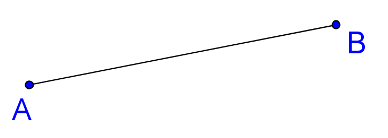Suppose that you have to find a point C on AB which divides AB in the ratio 2:1. This means that  $${\rm{AC:CB = 2:1}}$$ , as the following figure shows: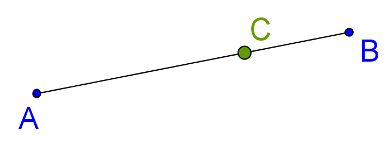How do we locate C? Before we move on, note that there is a huge difference between the following two statements:

• C divides AB in the ratio 2:1

• C divides BA in the ratio 2:1

The difference is that in the first case, the ratio of AC to BC is 2:1, while in the second case, the ratio of BC to AC is 2:1. Thus, the location of C will be entirely different in the two cases.

Now, back to the problem of how we can locate C. We will make use of the basic proportionality theorem. Through A, draw any ray AX, and cut off 3 equal intervals on it using a compass, as shown: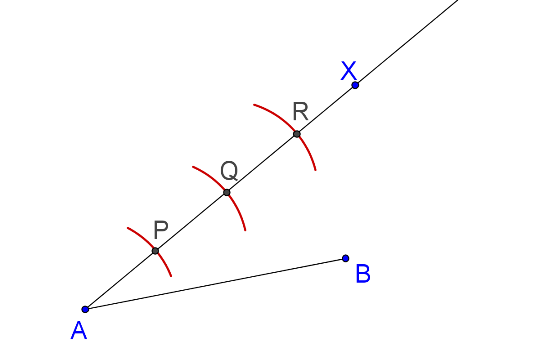Now, join R to B, and through Q, draw $${\rm{QZ || RB}}$$ . The point where QZ intersects AB is the point C: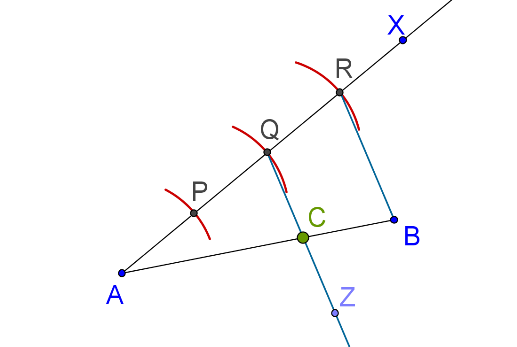Why does this work? Since $${\rm{QC || RB}}$$ , we have (using the BPT):

AC:CB = AQ:QR

But $${\rm{AQ:QR}}$$ is obviously 2:1, because we have cut off equal intervals on AX. Thus,

AC:CB = 2:1

Now, let us revisit this problem of internal division from the perspective of coordinates. Suppose that you are given the coordinates of two points A and B in the plane. How can you find the coordinates of a point C which divides AB internally in a given ratio? Note that we want to find the coordinates of C, that is, we want an algebraic answer, in terms of the coordinates of A and B.

Suppose that the coordinates of A and B are:

$\begin{array}{l}A \equiv \left( {{x_1},\;{y_1}} \right)\\B \equiv \left( {{x_2},\;{y_2}} \right)\end{array}$

We want to find a point C which divides AB internally in the ratio m:n. Let C be the point

$C \equiv \left( {h,\;k} \right)$

Consider the following figure: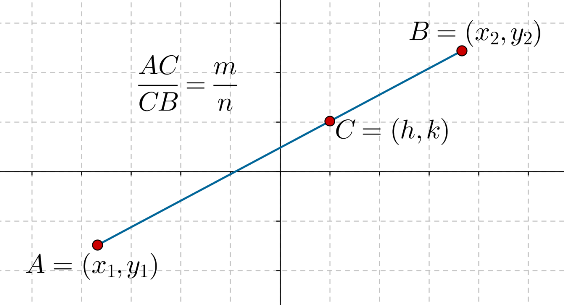Our problem is to find the coordinates of C in terms of the coordinates of A and B, and the parameters m and n. Let us complete the right triangle APB and AQC, as shown below: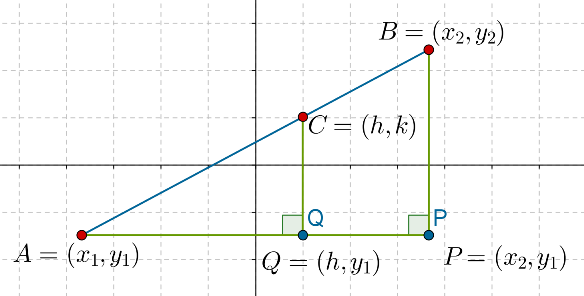Note that AQ and AP are parallel to the x axis, while BP and CQ are parallel to the y axis, and so:

$\begin{array}{l}P \equiv \left( {{x_2},\;{y_1}} \right)\\Q \equiv \left( {h,\;{y_1}} \right)\end{array}$

Now, we make the following observations:

\begin{align}&AP = {x_2} - {x_1},\;BP = {y_2} - {y_1}\\&AQ = h - {x_1},\;CQ = k - {y_1}\end{align}

Since  $$\Delta AQC{\rm{ }} \sim {\rm{ }}\Delta APB$$ , we have:

$\frac{{AQ}}{{AP}} = \frac{{CQ}}{{BP}} = \frac{{AC}}{{AB}} \;\;\;\;\;\;\;\;\;\;\;\;\;\;...({\rm{1}})$

Now comes the crucial part. We already have the expressions for the terms in the first two ratios above, but what about the third ratio? What is the value of$${\rm{AC:AB}}$$ ? Since C divides AB internally in the ratio m:n, we have:

\begin{align}& \frac{{AC}}{{CB}} = \frac{m}{n}\\& \Rightarrow \frac{{AC}}{{AB}} = \frac{m}{{m + n}}\end{align}

Make sure you understand how this is obtained. Using this value for $${\rm{AC:AB}}$$ in (1), we have:

\begin{align}& \frac{{AQ}}{{AP}} = \frac{{CQ}}{{BP}} = \frac{m}{{m + n}}\\ \Rightarrow & \frac{{h - {x_1}}}{{{x_2} - {x_1}}} = \frac{{k - {y_1}}}{{{y_2} - {y_1}}} = \frac{m}{{m + n}}\\ \Rightarrow & \left\{ \begin{gathered}\frac{{h - {x_1}}}{{{x_2} - {x_1}}} = \frac{m}{{m + n}}\\\frac{{k - {y_1}}}{{{y_2} - {y_1}}} = \frac{m}{{m + n}}\end{gathered} \right.\\ \Rightarrow & \left\{ \begin{gathered}h - {x_1} = \left( {\frac{m}{{m + n}}} \right)\left( {{x_2} - {x_1}} \right)\\k - {y_1} = \left( {\frac{m}{{m + n}}} \right)\left( {{y_2} - {y_1}} \right)\end{gathered} \right.\\ \Rightarrow & \left\{ \begin{gathered}h = {x_1} + \left( {\frac{m}{{m + n}}} \right)\left( {{x_2} - {x_1}} \right)\\k = {y_1} + \left( {\frac{m}{{m + n}}} \right)\left( {{y_2} - {y_1}} \right)\end{gathered} \right.\\ \Rightarrow & \left\{ \begin{gathered}h = \frac{{m{x_1} + n{x_1} + m{x_2} - m{x_1}}}{{m + n}}\\k = \frac{{m{y_1} + n{y_1} + m{y_2} - m{y_1}}}{{m + n}}\end{gathered} \right. \end{align}

$$\Rightarrow$$ $$\fbox{\displaystyle{{h\,\,\, = \frac{{m{x_2} + n{x_1}}}{{m + n}},\;k = \frac{{m{y_2} + n{y_1}}}{{m + n}}}}}$$

This is called the section formula, and it gives us the coordinates of C in terms of the coordinates of A and B, and the parameters m and n. Lets apply this formula to some examples.

Example-1: Consider the following two points:

$A = \left( { - 1,\;2} \right),\;B = \left( {2,\; - 3} \right)$

Find the point which divides AB internally in the ratio:

• 1:3

• 3:1

Solution: Let

$C = \left( {{x_C},\;{y_C}} \right),\;D = \left( {{x_D},\;{y_D}} \right)$

be the points which divide AB internally in the ratio 1:3 and 3:1 respectively. We now make use of the section formula.

### Coordinates of C

\begin{align}&{x_C} = \frac{{\underbrace {1 \times 2}_{m{x_2}} + \underbrace {3 \times - 1}_{n{x_1}}}}{{\underbrace {1 \times 3}_{m + n}}}\; = \frac{{2 - 3}}{4} = - \frac{1}{4}\\&{y_C} = \frac{{\underbrace {1 \times - 3}_{m{y_2}} + \underbrace {3 \times 2}_{n{y_1}}}}{{\underbrace {1 \times 3}_{m + n}}} = \frac{{ - 3 + 6}}{4} = \frac{3}{4}\\&\Rightarrow \;\;\;\;C = \left( {{x_C},\;{y_C}} \right) = \left( { - \frac{1}{4},\;\frac{3}{4}} \right)\end{align}

### Coordinates of D

\begin{align}&{x_D} = \frac{{\underbrace {3 \times 2}_{m{x_2}} + \underbrace {1 \times - 1}_{n{x_1}}}}{{\underbrace {3 \times 1}_{m + n}}} = \frac{{6 - 1}}{4} = \frac{5}{4}\\&{y_D}\, = \frac{{\underbrace {3 \times - 3}_{m{y_2}} + \underbrace {1 \times 2}_{n{y_1}}}}{{\underbrace {3 + 1}_{m + n}}}\; = \frac{{ - 9 + 2}}{4} = - \frac{7}{4}\\&\Rightarrow\;\;\;\;D = \left( {{x_D},\;{y_D}} \right) = \left( {\frac{5}{4},\; - \frac{7}{4}} \right)\end{align}

These two points are plotted on AB in the figure below: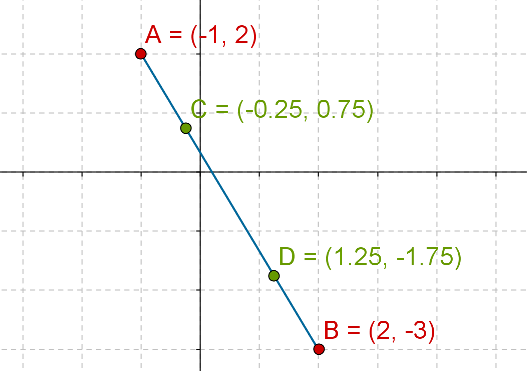Coordinate Geometry
Grade 9 | Questions Set 1
Coordinate Geometry
Coordinate Geometry
Grade 10 | Questions Set 1
Coordinate Geometry